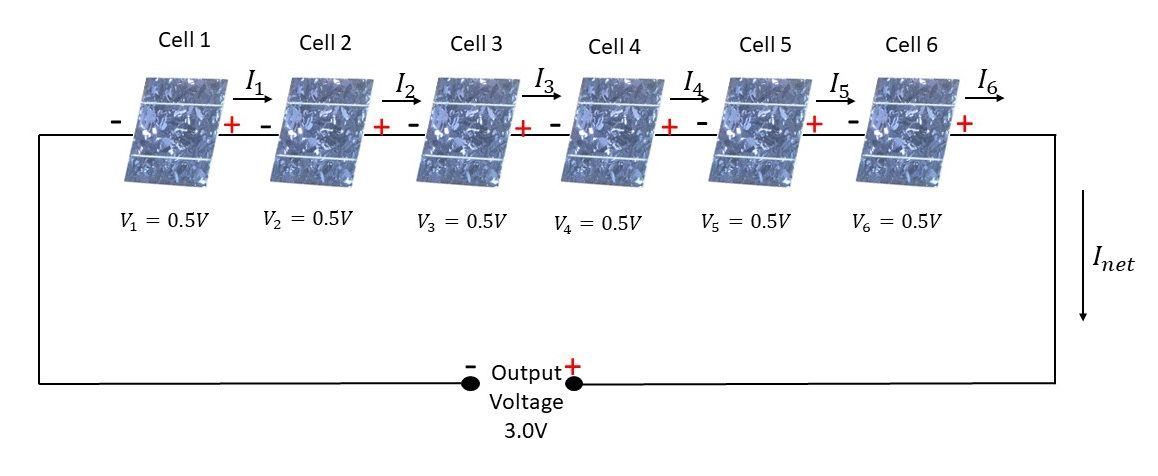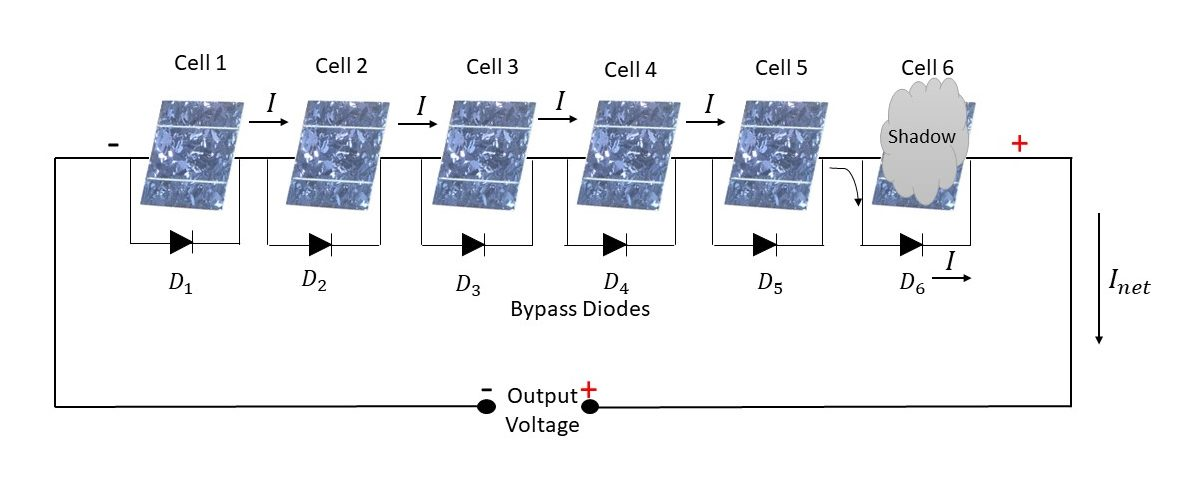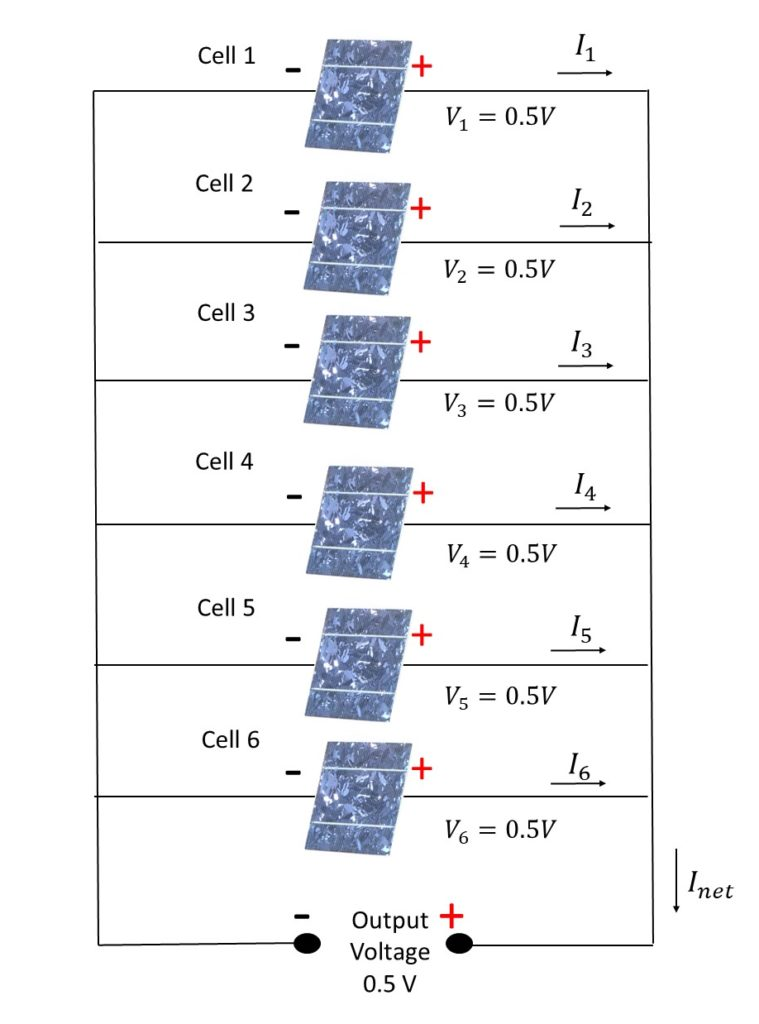# Solar PV Panel-Connection of Solar Cells

In our previous post on Solar PV Panel, we read about what it takes to make a solar panel, why we need to make a solar panel and how we make a solar panel from the solar cells. In this post we’ll dive into the details of different kind of connection of Solar Cells to form a Solar PV Panel as discussed in the last post.

So to begin with, Solar Cells are either connected in series or in parallel or combination of series-parallel to obtain the desired rating of voltage, current and power.

### Series Connection of Solar Cells

Series connected solar cells have the same current flowing through them as they all are in the same path for current to flow. Solar PV Panels consists of multiple solar cells which are connected together in series and are enclosed in a weather proof casing. This arrangement results in a single Solar PV Panel with higher voltage output as compared to a single Solar Cell as shown in the figure below.In the figure shown above, six solar cells are connected in series. As we saw in our earlier post, here also we’ll go with the assumption that the output voltage produced by an individual solar cell is 0.5 volts. Thus the combined output voltage in this case is the sum of the output voltages of the individual solar cells and is calculated as:

Output Voltage =𝑉1+𝑉2+𝑉3+𝑉4+𝑉5+𝑉6 =0.5V+0.5V+0.5V+0.5V+0.5V+0.5V = 3.0V

The combined output current in a series connected combination is same as the current produced by individual cells.

𝐼𝑛𝑒𝑡 = 𝐼1= 𝐼2 = 𝐼3 = 𝐼4 = 𝐼5 = 𝐼6

The combined power is the sum of power of individual cells or the net output voltage times the net current.

However there is one drawback to the series connection of solar cells. This is the case when there is a current mismatch among the connected cells. Even if one solar cell gets faulty or is partially or fully shaded from the sunlight, all the solar cells gets affected simulating a condition as if all the solar cells are shaded and thus resulting in loss of power output. There is also a possibility that the full current from rest of the solar cells is passing through the shaded or faulty solar cell causing the overheating and forming the “hotspot”. Thus it is really important to avoid shading on the PV Panels

To counter this effect when the performance of the entire panel goes down or shading results in formation of “hotspots” even when a single cell is shaded or gets faulty, Bypass Diodes are connected in parallel to each solar cell. The bypass diode is used to limit the voltage across the faulty/shaded cell in its reverse bias to pass a definite amount of current. The bypass diode conducts and thus allow the current to flow in the external circuit as shown in the figure below. The maximum reverse over voltage across the faulty/shaded cell is reduced to about a single diode drop so that the larger voltage differences cannot arise in the reverse-current direction across the cell, thus limiting the current flow and preventing the overheating due to dissipation of less power.### Parallel Connection of Solar Cells

Parallel Connected Solar Cells have the same voltage across all the cells in the circuit as the terminals of one cell is connected respectively to the terminals of the other cells.In the figure shown above, six solar cells are connected in parallel. The combined output voltage for the parallel connected cells is same and is equal to the voltage of the individual solar cells.

Output Voltage =𝑉1=𝑉2=𝑉3=𝑉4=𝑉5=𝑉6 = 0.5V

As we saw in our earlier post, here also we’ll go with the assumption that the output current produced by an individual solar cell is 3.0 amperes. Thus the combined output current in this case is the sum of output current of individual solar cells and is calculated as:

𝐼𝑛𝑒𝑡 = 𝐼1+ 𝐼2 + 𝐼3 + 𝐼4 + 𝐼5 + 𝐼6 = 3.0A+3.0A+3.0A+3.0A+3.0A+3.0A = 18A

The combined power is the sum of power of individual cells or the net output voltage times the net current.

As we have seen the impact of shading in case of series connection of solar cells, the parallel connection of solar cells is less sensitive to this type of shading mismatch. Though similar to the problem of current mismatch in case of series connection, in parallel connection of solar cells we have voltage mismatch when the cells are not identical.  This mismatch of voltage is generally not a problem when the net current output is low however as the net current increases, the damaged cell stops generating power and starts dissipating power thus reducing the output voltage of the solar panel.